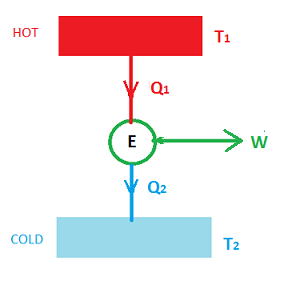# An innovative power cycle is being designed to operate between heat reservoirs at 150 degrees C...

## Question:

An innovative power cycle is being designed to operate between heat reservoirs at 150 degrees C and 55 degrees C. What is most nearly its maximum theoretical thermal efficiency?

## Heat Engine :

A Heat engine is a device which helps us to get an amount of work when connected to two thermal reservoirs, one at a higher temperature and another one at a lower temperature.In the above diagram, we can see that two thermal reservoirs one at higher temperature {eq}T_1 {/eq} and at a lower temperature {eq}T_2 {/eq} are connected such that heat flows between them.

The maximum efficiency of the heat engine is given as

{eq}\eta=\displaystyle \frac {T_1-T_2}{T_1} {/eq}

Given:

• Temperature of the heat source is {eq}T_1=150^oC=423 \ K {/eq}
• Temperature of the heat sink is {eq}T_2=55^oC=328 \ K {/eq}

The maximum theoretical thermal efficiency of the heat engine is given by

{eq}\begin{align*} \eta & = \frac {T_1-T_2}{T_1} \\ & = \frac {423-328}{423} \\ &=0.22 \Rightarrow(Answer) \end{align*} {/eq}